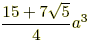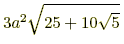index: click on a letter A B C D E F G H I J K L M N O P Q R S T U V W X Y Z A to Z index index: subject areas numbers & symbols sets, logic, proofs geometry algebra trigonometry advanced algebra & pre-calculus calculus advanced topics probability & statistics real world applications multimedia entrieswww.mathwords.com about mathwords website feedback

Dodecahedron
Regular Dodecahedron

A polyhedron with 12 faces. A regular dodecahedron has faces that are all regular pentagons.

Note: It is one of the five platonic solids.

 Regular Dodecahedron a = length of an edge Volume =Surface Area =Rotate me if your browser is Java-enabled.